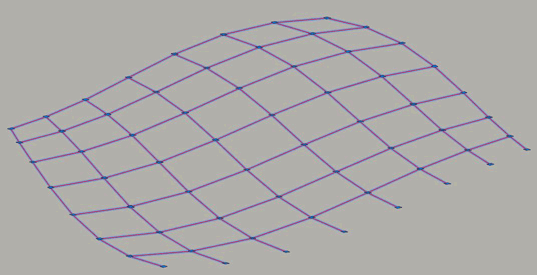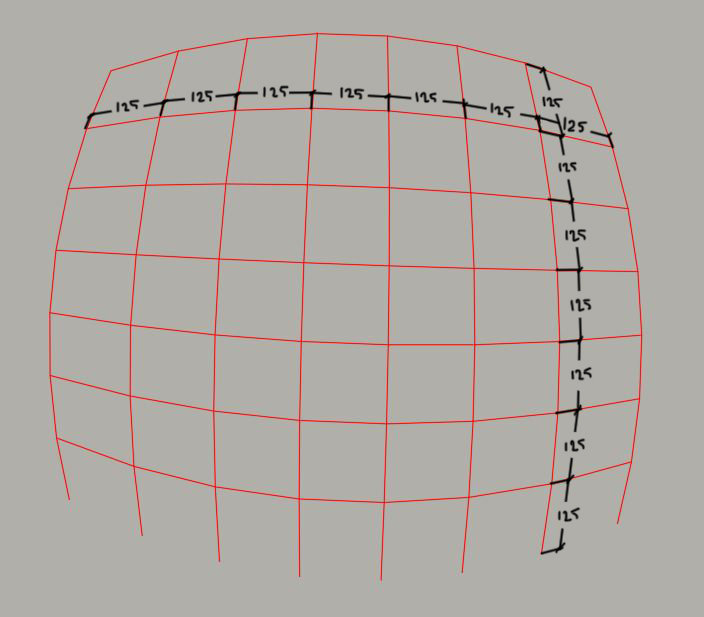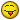# 3d Polyline Measuring

## Recommended PostsHi guys,

I have 3d polyline net system as you can see down below,trying to measure every cell automatically (see attachment),problem is that net is 3 dimensional and hard to define measuring angular, every 3d polyline is individual (see attachment)

just want to select the 3d polyline and giving measures between nodes, do anyone know how to solve this puzzle an easiest way?

Best Regards

##### Share on other sites33 minutes ago, syhdesign said:

problem is that net is 3 dimensional and hard to define measuring angular, every 3d polyline is individual (see attachment)

What attachment?Post a sample drawing, (AutoCAD 2010) in my case.

##### Share on other sites13 minutes ago, dlanorh said:

What attachment?Post a sample drawing, (AutoCAD 2010) in my case.

Here we go..

##### Share on other sitesGetting the distances between each polyline vertex is simple using vlax-curve functions, the question is how do you want them displayed (to include any relevant text heights, styles etc)?

##### Share on other sites42 minutes ago, dlanorh said:

Getting the distances between each polyline vertex is simple using vlax-curve functions, the question is how do you want them displayed (to include any relevant text heights, styles etc)?

Here display sample which i need to fill whole cells as shown in sample, each time I need to change UCS for every single mesh (cell) how can I automate this?

##### Share on other sitesTry this. It only inserts a text object at the mid point between vertices. The text is in the current layer and displays the distance between each vertex. When prompted select all the polylines. the variable t_ht controls the height of the text.

```(defun c:polydist ( / c_doc c_spc t_ht ss ent e_p s_p dist ang t_pt t_obj)

c_spc (vlax-get-property c_doc (if (= 1 (getvar 'cvport)) 'paperspace 'modelspace))
t_ht 20
);end_setq

(prompt "Select Polylines : ")
(setq ss (ssget '((0 . "*POLYLINE"))))
(repeat (setq cnt (sslength ss))
(setq ent (ssname ss (setq cnt (1- cnt)))
e_p (vlax-curve-getendparam ent)
s_p 0.0
)
(while (< s_p e_p)
(setq dist (- (vlax-curve-getdistatparam ent (1+ s_p)) (vlax-curve-getdistatparam ent s_p))
ang (angle '(0.0 0.0 0.0) (vlax-curve-getfirstderiv ent (/ (+ s_p s_p 1) 2.0)))
t_pt (vlax-curve-getpointatparam ent (/ (+ s_p (1+ s_p)) 2.0))
t_obj (vla-addtext c_spc (rtos dist 2 0) (vlax-3d-point t_pt) t_ht)
s_p (1+ s_p)
)
(mapcar '(lambda (x y) (vlax-put-property t_obj x y)) (list 'alignment 'textalignmentpoint 'rotation) (list acAlignmentBottomCenter (vlax-3d-point t_pt) ang))
)
)
)
```

It should do all selected polylines and give you an idea of how it can be automated.

•1

##### Share on other sites15 hours ago, dlanorh said:

Try this. It only inserts a text object at the mid point between vertices. The text is in the current layer and displays the distance between each vertex. When prompted select all the polylines. the variable t_ht controls the height of the text.

```
(defun c:polydist ( / c_doc c_spc t_ht ss ent e_p s_p dist ang t_pt t_obj)

c_spc (vlax-get-property c_doc (if (= 1 (getvar 'cvport)) 'paperspace 'modelspace))
t_ht 20
);end_setq

(prompt "Select Polylines : ")
(setq ss (ssget '((0 . "*POLYLINE"))))
(repeat (setq cnt (sslength ss))
(setq ent (ssname ss (setq cnt (1- cnt)))
e_p (vlax-curve-getendparam ent)
s_p 0.0
)
(while (< s_p e_p)
(setq dist (- (vlax-curve-getdistatparam ent (1+ s_p)) (vlax-curve-getdistatparam ent s_p))
ang (angle '(0.0 0.0 0.0) (vlax-curve-getfirstderiv ent (/ (+ s_p s_p 1) 2.0)))
t_pt (vlax-curve-getpointatparam ent (/ (+ s_p (1+ s_p)) 2.0))
t_obj (vla-addtext c_spc (rtos dist 2 0) (vlax-3d-point t_pt) t_ht)
s_p (1+ s_p)
)
(mapcar '(lambda (x y) (vlax-put-property t_obj x y)) (list 'alignment 'textalignmentpoint 'rotation) (list acAlignmentBottomCenter (vlax-3d-point t_pt) ang))
)
)
)
```

It should do all selected polylines and give you an idea of how it can be automated.

working like charm, thank you very much, great stuff

## Join the conversation

You can post now and register later. If you have an account, sign in now to post with your account.
Note: Your post will require moderator approval before it will be visible.×   Pasted as rich text.   Paste as plain text instead

Only 75 emoji are allowed.